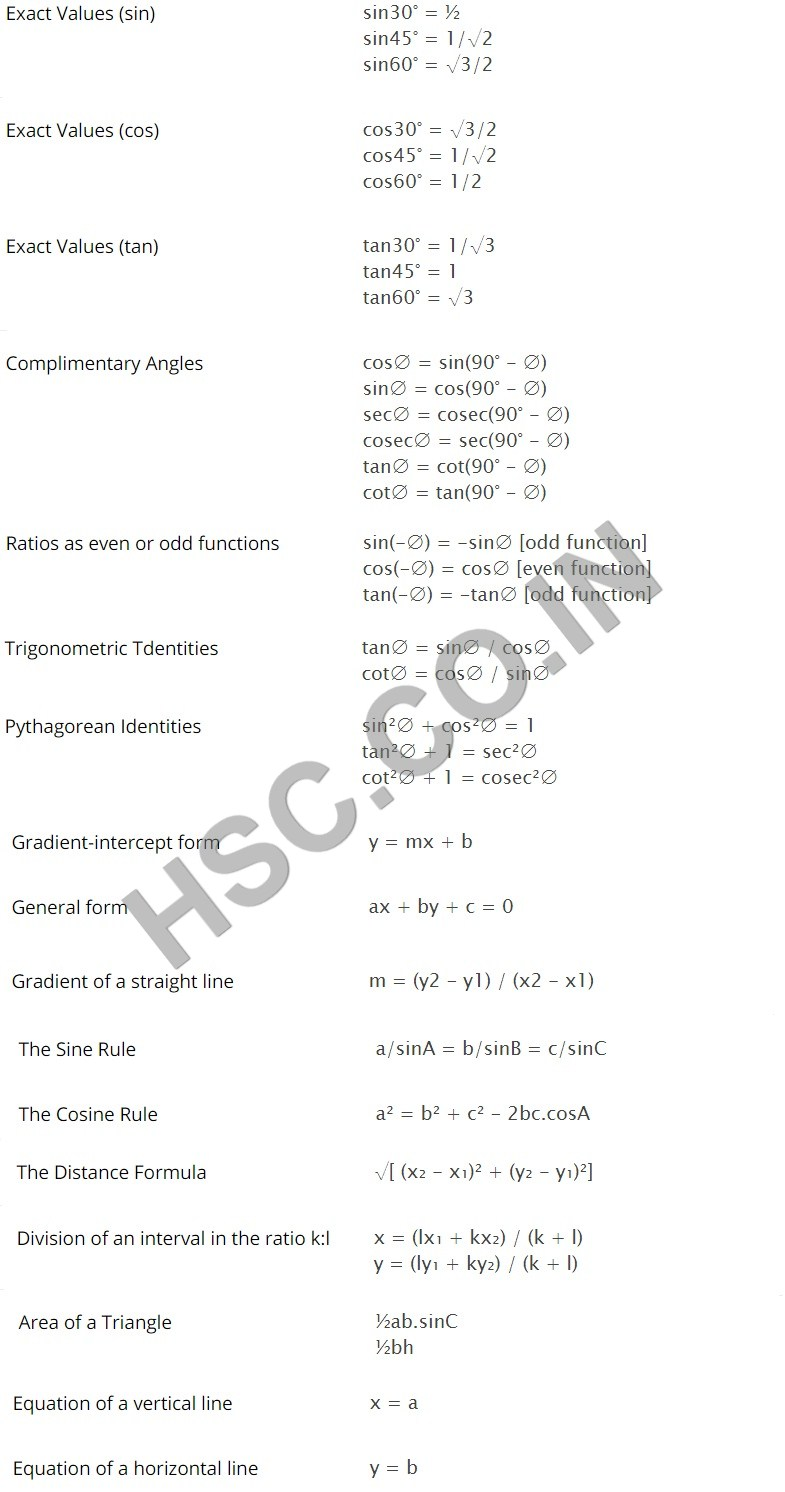# Maths Formulas – HSC Board

## General Maths formulas for class 12th HSC Board

### List – class 12th General Maths of formulas:

The Quadratic formula                                     x = [-b ±  √(b2 – 4ac)] / 2a

Expansions                                                            (a = b)2 = a2 + 2ab + b2

Equation of a Circle                                           (x – a)2 + (Y – b2) = r2

Equation of a semi Circel                                y = b ±  √(r2 – (x – a)2)

Sums and Differences

of Cubcs                                                                  x3 + y3 = (x + y)(x2 – xy + y2)

x3 – y3 = (x-y)(x2 + xy + y2)

Axis of symetry                                                  x = -b/2a

Trigonometric Ratios                                    sin α  = O/H

cos α  = A/H

tanα = O/A

Reciprocal Ratios                                          cosec α = 1/ sin α  = O/H

sec α = 1/ cos α = H/A

cot α = 1/ tanα = O/A